# JP5651990B2 - Digital coherent receiver and receiving method - Google Patents

## Info

Publication number
JP5651990B2
JP5651990B2 JP2010094436A JP2010094436A JP5651990B2 JP 5651990 B2 JP5651990 B2 JP 5651990B2 JP 2010094436 A JP2010094436 A JP 2010094436A JP 2010094436 A JP2010094436 A JP 2010094436A JP 5651990 B2 JP5651990 B2 JP 5651990B2
Authority
JP
Japan
Prior art keywords
unit
frequency offset
digital signal
spectrum
signal converted
Prior art date
Legal status (The legal status is an assumption and is not a legal conclusion. Google has not performed a legal analysis and makes no representation as to the accuracy of the status listed.)
Active
Application number
JP2010094436A
Other languages
Japanese (ja)
Other versions
JP2011228819A (en
Inventor

Original Assignee

Priority date (The priority date is an assumption and is not a legal conclusion. Google has not performed a legal analysis and makes no representation as to the accuracy of the date listed.)
Filing date
Publication date
Application filed by 富士通株式会社 filed Critical 富士通株式会社
Priority to JP2010094436A priority Critical patent/JP5651990B2/en
Publication of JP2011228819A publication Critical patent/JP2011228819A/en
Application granted granted Critical
Publication of JP5651990B2 publication Critical patent/JP5651990B2/en
Active legal-status Critical Current
Anticipated expiration legal-status Critical

• 230000001427 coherent Effects 0 title claims description 45
• 238000001228 spectrum Methods 0 claims description 107
• 238000006243 chemical reaction Methods 0 claims description 46
• 230000001276 controlling effects Effects 0 claims description 3
• 238000000034 methods Methods 0 description 5
• 238000005070 sampling Methods 0 description 5
• 230000001934 delay Effects 0 description 3
• 230000000694 effects Effects 0 description 1

## Images

•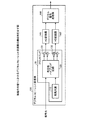•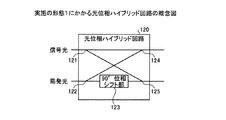•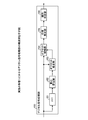•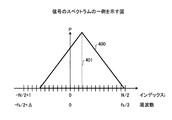•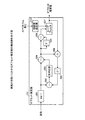•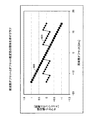•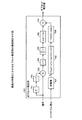•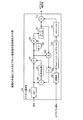•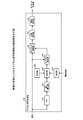•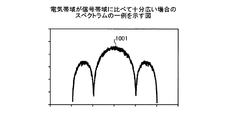•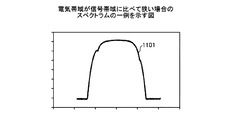•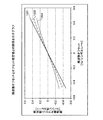•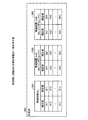•## Classifications

• HELECTRICITY
• H04ELECTRIC COMMUNICATION TECHNIQUE
• H04BTRANSMISSION
• H04B10/00Transmission systems employing electromagnetic waves other than radio-waves, e.g. infrared, visible or ultraviolet light, or employing corpuscular radiation, e.g. quantum communication
• H04B10/616Details of the electronic signal processing in coherent optical receivers
• H04B10/6164Estimation or correction of the frequency offset between the received optical signal and the optical local oscillator
• HELECTRICITY
• H04ELECTRIC COMMUNICATION TECHNIQUE
• H04BTRANSMISSION
• H04B10/00Transmission systems employing electromagnetic waves other than radio-waves, e.g. infrared, visible or ultraviolet light, or employing corpuscular radiation, e.g. quantum communication
• H04B10/65Intradyne, i.e. coherent receivers with a free running local oscillator having a frequency close but not phase-locked to the carrier signal
• HELECTRICITY
• H04ELECTRIC COMMUNICATION TECHNIQUE
• H04LTRANSMISSION OF DIGITAL INFORMATION, e.g. TELEGRAPHIC COMMUNICATION
• H04L27/00Modulated-carrier systems
• H04L27/18Phase-modulated carrier systems, i.e. using phase-shift keying includes continuous phase systems
• H04L27/223Demodulation in the optical domain

## Description

The present invention relates to a digital coherent receiver and a receiving method for receiving light.

Due to the increase in traffic on the Internet, there is a need to increase the capacity of trunk optical communication systems, and research and development of optical transceivers capable of transmitting signals exceeding 100 [Gbit / s] per wavelength are being conducted. . When the bit rate per wavelength is increased, the OSNR (Optical Signal Noise Ratio) strength is reduced, and the signal quality is degraded due to waveform distortion due to wavelength dispersion, polarization mode dispersion, or non-linear effects in the transmission path. Becomes larger.

For this reason, in recent years, a digital coherent reception method having an OSNR tolerance and a waveform distortion tolerance of a transmission path has been studied. In contrast to the conventional method of directly detecting on / off of the light intensity by assigning the binary signal to the binary signal, in the digital coherent reception method, the light intensity and the phase information are extracted by the coherent reception method. The extracted intensity and phase information is quantized by an AD (Analog / Digital) converter, and demodulated by a digital signal processing unit.

One advantage of the digital coherent receiver is that a mechanism for synchronizing the frequency and phase of the transmission light source and the local light source with respect to the received light can be implemented as digital signal processing. This makes it possible to synchronize the frequency and phase of the transmission light source and the local transmission light source with respect to the reception light without mounting an optical PLL (Phase Locked Loop) that is difficult to realize.

When a general wavelength multiplexing light source is used, an optical frequency shift (frequency offset) occurs within the range of wavelength accuracy between the light source on the transmitter side and the LO light source (Local Oscillator) on the receiver side. Can do. In the case of a general wavelength multiplexing light source, there is a possibility that this frequency offset is several [GHz] at the maximum.

As an example, a case where a QPSK (Quadrature Phase Shift Keying) signal is received will be described. When the frequency offset is sufficiently small, only the static rotation due to the phase shift is observed in the signal constellation observed over a short time. On the other hand, when the frequency offset is large, since the constellation rotates within the observation time due to the frequency offset, it becomes difficult to process and identify the QPSK signal as it is. For this reason, frequency offset compensation is used. Hereinafter, not only the frequency difference between the transmission light source and the LO light source, but also the phase rotation caused by the frequency offset is called a frequency offset.

In order to compensate for the frequency offset, for example, the frequency offset is estimated by an offset estimation circuit, and the constellation is reversely rotated in the digital circuit by the estimated frequency offset. As an example of an offset estimation circuit, a technique is known in which, for example, an m-value PSK signal is raised to the power of m to remove a coding component and a noise component from complex electric field information and extract a frequency offset component (for example, (See Non-Patent Document 1 below.) In addition, a technique is known that performs provisional determination of a signal, subtracts the result of the provisional determination to remove an encoded component, and expands an estimated range of a frequency offset (see, for example, Non-Patent Document 2 below).

A. Leven et al. "Frequency Estimates in Intradyne Reception", IEEE Photonics Technology Letters, Vol. 19, no. 6, p. 566-368, March 2007 H. Nakashima et al. "Novel Wide-range Frequency Offset Compensator Evaluated with Real-time Digital Coherent Receiver", 34th European Conference and Exhibitation of the Co-op. 3. D. 4, September 2008

However, in the above-described conventional technique, the frequency offset estimation circuit has a limit of the pull-in range, and the frequency offset outside the pull-in range cannot be accurately estimated. For this reason, there is a problem that the frequency offset cannot be accurately compensated and the reception quality is lowered. On the other hand, it is conceivable to use differential decoding, but if differential decoding is used, there is a problem in that reception noise resistance is reduced.

The disclosed digital coherent receiver and reception method are intended to solve the above-described problems and to improve reception quality.

In order to solve the above-described problems and achieve the object, the disclosed technology includes a front end that performs photoelectric conversion using signal light and local light, a conversion unit that converts an output of the front end into a digital signal, and the conversion A calculation unit that calculates a spectrum centroid of the digital signal converted by the unit, an estimation unit that estimates a frequency offset of the digital signal based on the spectrum centroid calculated by the calculation unit, and a frequency estimated by the estimation unit And an adjustment unit that reduces the frequency offset of the digital signal based on the offset.

According to the disclosed digital coherent receiver and reception method, the reception quality can be improved.

FIG. 3 is a diagram illustrating a configuration example of a digital coherent receiver according to the first exemplary embodiment; 1 is a conceptual diagram of an optical phase hybrid circuit according to a first exemplary embodiment; FIG. 2 is a diagram illustrating a configuration example of a digital signal processing unit according to the first embodiment. It is a figure which shows an example of the spectrum of a signal. 3 is a diagram illustrating a configuration example of an offset estimation unit according to the first embodiment; FIG. It is a graph which shows the relationship between a frequency offset and an offset estimated value. It is a figure which shows the structural example of the offset estimation part concerning Embodiment 2. FIG. It is a figure which shows the modification of the offset estimation part concerning Embodiment 2. FIG. FIG. 6 is a diagram illustrating a configuration example of a digital signal processing unit according to a third embodiment. It is a figure which shows an example of a spectrum in case an electrical band is sufficiently wide compared with a signal band. It is a figure which shows an example of a spectrum in case an electrical band is narrow compared with a signal band. It is a graph which shows the relationship between a frequency offset and an offset estimated value. It is a figure which shows an example of the correction information memorize | stored in a memory | storage part. FIG. 6 is a diagram illustrating a configuration example of a digital signal processing unit according to a fourth embodiment.

Hereinafter, preferred embodiments of the disclosed technology will be described in detail with reference to the accompanying drawings. The disclosed technique uses a spectrum centroid of a digitally converted signal in a digital coherent receiver, thereby accurately compensating for a frequency offset between signal light and local light and improving reception quality.

(Embodiment 1)
FIG. 1 is a diagram illustrating a configuration example of a digital coherent receiver according to the first embodiment. As shown in FIG. 1, the digital coherent receiver 100 according to the first embodiment includes a local light source 110, an optical phase hybrid circuit 120, photoelectric conversion elements 131 and 132, AD converters 141 and 142, and a digital signal. A signal processing unit 150. The digital coherent receiver 100 receives QPSK signal light, for example.

The front end 10 including the local light source 110, the optical phase hybrid circuit 120, and the photoelectric conversion elements 131 and 132 is a front end that performs photoelectric conversion by mixing signal light received by the digital coherent receiver 100 and local light. is there. Specifically, the local light source 110 generates local light and outputs the generated local light to the optical phase hybrid circuit 120. The local light source 110 is, for example, ITLA.

The optical phase hybrid circuit 120 extracts a complex electric field indicating the light intensity and phase of the optical signal by mixing the signal light and the local light output from the local light source 110. The optical phase hybrid circuit 120 outputs light (I signal) having an intensity corresponding to the real part of the extracted complex electric field to the photoelectric conversion element 131. The optical phase hybrid circuit 120 outputs light (Q signal) having an intensity corresponding to the imaginary part of the extracted complex electric field to the photoelectric conversion element 132. The configuration of the optical phase hybrid circuit 120 will be described later with reference to FIG.

The photoelectric conversion element 131 photoelectrically converts the optical I signal output from the optical phase hybrid circuit 120, and outputs the photoelectrically converted I signal to the AD converter 141. The photoelectric conversion element 132 photoelectrically converts the Q signal output from the optical phase hybrid circuit 120 and outputs the photoelectrically converted Q signal to the AD converter 142. Each of the photoelectric conversion element 131 and the photoelectric conversion element 132 is, for example, a photodiode (PD: Photo Diode).

The AD converters 141 and 142 are conversion units that convert the output of the front end 10 into a digital signal. Specifically, the AD converter 141 converts the I signal output from the photoelectric conversion element 131 into a digital signal by sampling at a predetermined sampling frequency. The AD converter 142 converts the Q signal output from the photoelectric conversion element 132 into a digital signal by sampling at a predetermined sampling frequency. Each of the AD converters 141 and 142 outputs a signal converted into a digital signal to the digital signal processing unit 150.

The digital signal processing unit 150 identifies signal light by performing digital processing on the I signal and the Q signal output from the AD converters 141 and 142. The digital signal processing unit 150 outputs a signal light identification result. The configuration of the digital signal processing unit 150 will be described later with reference to FIG. 3, for example.

FIG. 2 is a conceptual diagram of the optical phase hybrid circuit according to the first embodiment. As shown in FIG. 2, the optical phase hybrid circuit 120 includes branch parts 121 and 122, a 90 ° phase shift part 123, and interference parts 124 and 125. An optical signal is input to the branching unit 121. The branching unit 121 branches the input signal light and outputs the branched signal light to the interference unit 124 and the interference unit 125, respectively.

The local light from the local light source 110 is input to the branching unit 122. The branching unit 122 branches the input local light and outputs the branched local light to the interference unit 124 and the 90 ° phase shift unit 123, respectively. The 90 ° phase shift unit 123 shifts the phase of local light output from the branching unit 122 by 90 °. The 90 ° phase shift unit 123 outputs the local light whose phase is shifted to the interference unit 125.

The interference unit 124 causes the signal light output from the branch unit 121 and the local light output from the branch unit 122 to interfere with each other. The interference unit 124 outputs the interfered light to the photoelectric conversion element 131 as an I signal. The interference unit 125 causes the signal light output from the branching unit 121 to interfere with the local light output from the 90 ° phase shift unit 123. The interference unit 125 outputs the interfered light to the photoelectric conversion element 132 as a Q signal.

FIG. 3 is a diagram of a configuration example of the digital signal processing unit according to the first embodiment. As shown in FIG. 3, the digital signal processing unit 150 includes a Fourier transform unit 151, a centroid calculation unit 152, an offset estimation unit 153, an offset compensation unit 154, a phase estimation unit 155, an identification determination unit 156, It has. For example, the digital signal processing unit 150 receives a complex signal having the I signal output from the AD converter 141 as a real part and the Q signal output from the AD converter 142 as an imaginary part.

The Fourier transform unit 151 and the centroid calculation unit 152 are calculation units that calculate the spectrum centroid of the signals converted by the AD converters 141 and 142. Specifically, the Fourier transform unit 151 performs a Fourier transform (FFT: Fast Fourier Transform) on the signal input to the digital signal processing unit 150. The Fourier transform unit 151 outputs the spectrum obtained by the Fourier transform to the centroid calculation unit 152.

The centroid calculation unit 152 calculates a spectrum centroid that is the centroid of the spectrum output from the Fourier transform unit 151. The spectrum centroid is, for example, the center frequency of the frequency range in which signals are distributed in the spectrum. The centroid calculation unit 152 calculates the spectrum centroid by, for example, the following equation (1).

In the above equation (1), i is an index indicating the frequency of the spectrum. P (i) is the power of the spectrum at the frequency indicated by index i. N is the FFT size of the Fourier transform in the Fourier transform unit 151. Δ is fs / (N−1). fs is a sampling frequency in the AD converters 141 and 142. fb is the baud rate of the signal light received by the digital coherent receiver 100. The centroid calculation unit 152 outputs the calculated spectrum centroid to the offset estimation unit 153.

The offset estimation unit 153 estimates the frequency offset of the signal input to the digital signal processing unit 150 based on the spectrum centroid output from the centroid calculation unit 152. The offset estimation unit 153 outputs an offset estimation value indicating the frequency offset estimation result to the offset compensation unit 154. The configuration of the offset estimation unit 153 will be described later with reference to FIG. 5, for example.

The offset compensation unit 154 (adjustment unit) compensates for the frequency offset of the signal input to the digital signal processing unit 150 based on the offset estimation value output from the offset estimation unit 153. For example, the offset compensation unit 154 compensates for the frequency offset of the signal by giving an inverse characteristic of the offset estimation value output from the offset estimation unit 153. However, the compensation of the frequency offset by the offset compensator 154 is not limited to the case where the frequency offset is set to 0, but may be anything that reduces the frequency offset. The offset compensation unit 154 outputs a signal compensated for the frequency offset to the phase estimation unit 155.

The phase estimation unit 155 estimates and compensates for the signal output from the offset compensation unit 154 by estimating the phase difference between the signal light received by the digital coherent receiver 100 and the local light of the local light source 110. To do. The phase estimation unit 155 outputs a signal that compensates for the phase difference to the identification determination unit 156. The identification determination unit 156 identifies the signal output from the phase estimation unit 155. The identification determination unit 156 outputs the identification result.

FIG. 4 is a diagram illustrating an example of a spectrum of a signal. The horizontal axis in FIG. 4 indicates the frequency and the index i. The index i is an index corresponding to the frequency. Specifically, −N / 2 + 1 to N / 2 of the index i correspond to the frequencies −fs / 2 + Δ to fs / 2, respectively. The vertical axis in FIG. 4 indicates the power P of the frequency component. A spectrum 400 is a spectrum output from the Fourier transform unit 151.

The spectrum centroid 401 is a spectrum centroid calculated by the centroid calculation unit 152. The spectrum centroid 401 deviates from 0 according to the frequency offset. Therefore, the offset estimation unit 153 can estimate the frequency offset efficiently by estimating the frequency offset based on the spectrum centroid 401.

FIG. 5 is a diagram of a configuration example of the offset estimation unit according to the first embodiment. As illustrated in FIG. 5, the offset estimation unit 153 includes a declination calculation unit 501, a subtraction unit 502, a temporary determination unit 503, a subtraction unit 504, a delay unit 505, a subtraction unit 506, and a loop filter 507. , An adder 508, a delay unit 509, and an initial value setting unit 510.

The offset estimation unit 153 illustrated in FIG. 5 recursively estimates the frequency offset of the input signal. That is, the offset estimation unit 153 estimates the frequency offset while feeding back the calculation result of the frequency offset (the output of the loop filter 507).

The signal input to the offset estimation unit 153 is input to the deflection angle calculation unit 501. The deflection angle calculation unit 501 calculates the phase angle indicated by the input complex signal. The deflection angle calculation unit 501 outputs a signal indicating the calculated phase angle to the subtraction unit 502 and the subtraction unit 504. The subtraction unit 502 subtracts the signal output from the delay unit 509 from the signal output from the deflection angle calculation unit 501. The subtraction unit 502 outputs the subtraction result to the temporary determination unit 503.

The temporary determination unit 503 performs a temporary determination (identification) on the signal based on the subtraction result output from the subtraction unit 502. The temporary determination unit 503 outputs a signal indicating the temporary determination result to the subtraction unit 504. The subtraction unit 504 subtracts the signal output from the temporary determination unit 503 from the signal output from the declination calculation unit 501. Subtraction unit 504 outputs a signal indicating the subtraction result to delay unit 505, subtraction unit 506, and addition unit 508.

The delay unit 505 delays the signal output from the subtraction unit 504 by one symbol. The delay unit 505 outputs the delayed signal to the subtraction unit 506. The subtraction unit 506 subtracts the signal output from the subtraction unit 504 from the signal output from the delay unit 505. The subtraction unit 506 outputs a signal indicating the subtraction result to the loop filter 507.

The loop filter 507 averages the signal output from the subtraction unit 506. For example, the loop filter 507 is an IIR (Infinite Impulse Response) filter having an infinite impulse response, for example. The loop filter 507 outputs the averaged signal as an offset estimated value. Further, the loop filter 507 outputs the averaged signal to the adding unit 508.

The adder 508 adds the signal output from the subtractor 504 and the signal output from the loop filter 507. Adder 508 outputs a signal indicating the addition result to delay unit 509. The delay unit 509 delays the signal output from the adder 508 by one symbol. The delay unit 509 outputs the delayed signal to the subtraction unit 502.

The initial value setting unit 510 sets the spectrum centroid output from the centroid calculation unit 152 as the initial value of the signal output from the loop filter 507. As described above, the offset estimation unit 153 recursively estimates the frequency offset of the input signal using the spectrum centroid calculated by the centroid calculation unit 152 as an initial value.

The signal at time t + 1 output from the deflection angle calculation unit 501 indicates the phase angle θ (t + 1). The phase angle θ (t + 1) can be represented by θd (t + 1) + θfo (t + 1) + θPN (t + 1). θd (t + 1) indicates a modulation component. θfo (t + 1) indicates the amount of phase rotation caused by the frequency offset. θPN (t + 1) indicates phase noise.

Further, since the signal output from the temporary determination unit 503 indicates the temporary determination result of the modulation component, it is approximately θd (t + 1). For this reason, the signal output from the subtraction unit 504 can be represented by θfo (t + 1) + θPN (t + 1). The signal output from the delay unit 505 can be represented by θfo (t) + θPN (t).

Therefore, the signal output from the subtracting unit 506 can be represented by θfo (t + 1) −θfo (t) + θPN (t + 1) −θPN (t). Assuming that θPN (t + 1) = θPN (t), the signal output from the subtracting unit 506 can be expressed by Δfo = θfo (t + 1) −θfo (t). Since Δfo is a change amount of the phase rotation amount between symbols, it indicates a frequency offset. The loop filter 507 can obtain an offset estimated value by averaging the frequency offset Δfo.

Further, the signal output from the adding unit 508 is θfo (t + 1) + θPN (t + 1) + Δfo. Therefore, the signal output from the delay unit 509 is θfo (t) + θPN (t) + Δfo. Here, θfo (t) = θfo (t + 1) −Δfo. Further, it can be considered that θPN (t) = θPN (t + 1). For this reason, the signal output from the delay unit 509 is θfo (t + 1) + θPN (t + 1). Therefore, the signal output from the subtracting unit 502 is the modulation component θd (t + 1).

FIG. 6 is a graph showing the relationship between the frequency offset and the offset estimated value. In FIG. 6, the horizontal axis represents the actual frequency offset [GHz]. The vertical axis represents the estimated offset value output from the offset estimation unit 153. The relationship 601 shows the relationship between the actual frequency offset and the offset estimated value when the initial value of the output of the loop filter 507 is assumed to be a fixed value (for example, 0).

When the initial value of the output of the loop filter 507 is a fixed value (0 GHz in FIG. 6), if the difference between the initial value and the actual frequency offset becomes large, the phase change due to the frequency offset becomes a change in value due to phase modulation. Misunderstood. For this reason, the offset estimated value output from the offset estimation part 153 changes periodically with respect to an actual frequency offset. For this reason, the actual frequency offset cannot be accurately estimated.

A relationship 602 shows the characteristic of the offset estimated value with respect to the actual frequency offset when the spectrum gravity center is set as the initial value of the output of the loop filter 507. By setting the spectrum centroid to the initial value of the output of the loop filter 507, the initial value can always be within the pull-in range, so that an actual frequency offset is obtained from the offset estimated value output from the offset estimating unit 153. Can do.

Thus, according to the digital coherent receiver 100 according to the first embodiment, the frequency offset can be estimated using the spectrum centroid of the signal. Specifically, by setting the spectrum centroid of the signal as an initial value in the offset estimation unit 153 that recursively estimates the frequency offset, the frequency offset is accurately estimated regardless of the frequency offset at the time of reception of the signal light. (See, for example, relationship 602 in FIG. 6).

For this reason, it is possible to accurately compensate for the frequency offset and improve the reception quality. In addition, the frequency offset pull-in range can be expanded. For example, the frequency offset pull-in range can be expanded without using differential decoding. For this reason, it is possible to improve reception quality while suppressing a decrease in reception noise tolerance characteristics.

Further, by setting the spectrum centroid of the signal as an initial value in the offset estimation unit 153 that recursively estimates the frequency offset, the frequency offset estimation result can be converged in a short time. For this reason, the start-up time of the digital coherent receiver 100 can be shortened.

(Embodiment 2)
In the digital coherent receiver 100 according to the second embodiment, the description of the same parts as those of the digital coherent receiver 100 according to the first embodiment will be omitted.

FIG. 7 is a diagram of a configuration example of the offset estimation unit according to the second embodiment. As shown in FIG. 7, the offset estimation unit 153 of the digital coherent receiver 100 according to the second embodiment includes a delay unit 701, a complex conjugate calculation unit 702, a multiplication unit 703, a power unit 704, and an averaging unit. 705, a deflection angle calculation unit 706, a conversion unit 707, a quotient calculation unit 708, a calculation unit 709, and an addition unit 710. The offset estimation unit 153 illustrated in FIG. 7 estimates the frequency offset by correcting the frequency offset calculated based on the input signal based on the spectrum centroid calculated by the centroid calculation unit 152.

The signal (complex number) input to the offset estimation unit 153 is input to the delay unit 701 and the multiplication unit 703. Delay section 701 delays the input signal by one symbol, and outputs the delayed signal to complex conjugate calculation section 702. Complex conjugate calculation section 702 calculates the complex conjugate of the signal output from delay section 701, and outputs the calculated complex conjugate to multiplication section 703.

Multiplication section 703 multiplies the signal input to offset estimation section 153 and the complex conjugate output from complex conjugate calculation section 702. The multiplication result by the multiplication unit 703 indicates the phase difference (phase rotation amount) between symbols of the signal input to the offset estimation unit 153. However, the phase rotation amount indicated by the multiplication result by the multiplication unit 703 includes the phase rotation amount due to the modulation component of the signal and the phase rotation amount due to the frequency offset. Multiplier 703 outputs a signal indicating the multiplication result to power unit 704.

The power unit 704 multiplies the signal output from the multiplier 703 to the m-th power. m is the number of values indicated by one symbol of the signal. For example, when the signal modulation method is QPSK, the power unit 704 raises the signal to the fourth power. Thereby, the phase rotation amount due to the modulation component of the signal can be removed from the phase rotation amount indicated by the signal output from the multiplier 703. For this reason, the signal raised to the m-th power of the power unit 704 indicates the phase rotation amount due to the frequency offset (m times the actual phase rotation amount). The power unit 704 outputs the m-th power signal to the averaging unit 705.

The averaging unit 705 adds the signals output from the power unit 704 by N samples. Thereby, the noise of the signal output from the power unit 704 can be removed. The averaging unit 705 outputs a signal indicating the addition result to the declination calculation unit 706. The argument calculation unit 706 calculates the argument of the complex signal output from the averaging unit 705, and divides the calculated argument by m. Thereby, a frequency offset can be obtained. The deflection angle calculation unit 706 outputs the calculated frequency offset to the addition unit 710 as an offset estimated value.

The spectrum centroid output from the centroid calculation unit 152 is input to the conversion unit 707. The conversion unit 707 converts the input spectrum centroid into a phase rotation amount of one symbol time. The conversion unit 707 outputs the converted phase rotation amount x to the quotient calculation unit 708. The quotient calculation unit 708 calculates an integer part of a quotient obtained by dividing a value obtained by adding π / m to the phase rotation amount x output from the conversion unit 707 by 2π / m (FLOOR ((x + π / m) / (2π / M))). The quotient calculation unit 708 outputs a signal i indicating the calculation result to the calculation unit 709.

The calculation unit 709 calculates −1 * (2π / m) * i based on the signal i output from the quotient calculation unit 708. The calculating unit 709 outputs the calculation result to the adding unit 710 as a correction value for the offset estimated value. The adding unit 710 adds the offset estimated value output from the declination calculating unit 706 and the correction value output from the declination calculating unit 706. Adder 710 outputs an estimated offset value obtained by adding the correction value.

Thereby, the offset estimation part 153 can correct | amend the offset estimated value estimated based on the frequency offset estimated by a spectrum gravity center in m-value PSK. For this reason, even if the actual frequency offset becomes larger than the estimation range and the offset estimation value periodically changes with respect to the actual frequency offset (see, for example, the relationship 601 in FIG. 6), the frequency offset is accurately estimated. can do.

FIG. 8 is a diagram illustrating a modification of the offset estimation unit according to the second embodiment. In FIG. 8, the same components as those shown in FIGS. 5 and 7 are denoted by the same reference numerals and description thereof is omitted. As shown in FIG. 8, in addition to the configuration of the offset estimation unit 153 shown in FIG. 5, the offset estimation unit 153 includes a conversion unit 707, a quotient calculation unit 708, a calculation unit 709, and an addition unit 710. You may have. In this case, the initial value setting unit 510 shown in FIG. 5 may be omitted.

The loop filter 507 outputs the offset estimated value to the adding unit 710. Adder 710 adds the offset estimated value output from loop filter 507 and the correction value output from calculator 709. Thereby, the offset estimation part 153 can correct | amend the offset estimated value estimated based on the frequency offset estimated by a spectrum gravity center in m-value PSK.

Thus, according to the digital coherent receiver 100 according to the second embodiment, the frequency offset can be estimated using the spectrum centroid of the signal. Specifically, the frequency offset calculated based on the input signal is corrected based on the spectrum center of gravity of the signal, so that the frequency offset can be accurately estimated regardless of the frequency offset when receiving the signal light. Can do.

For this reason, it is possible to accurately compensate for the frequency offset and improve the reception quality. In addition, the frequency offset pull-in range can be expanded. For example, the frequency offset pull-in range can be expanded without using differential decoding. For this reason, it is possible to improve reception quality while suppressing a decrease in reception noise tolerance characteristics.

(Embodiment 3)
In the digital coherent receiver 100 according to the third embodiment, the description of the same parts as those of the digital coherent receiver 100 according to the first embodiment will be omitted.

FIG. 9 is a diagram of a configuration example of the digital signal processing unit according to the third embodiment. In FIG. 9, the same components as those shown in FIG. As illustrated in FIG. 9, the digital signal processing unit 150 according to the third embodiment includes a setting unit 901, a storage unit 902, and a correction unit 903 in addition to the configuration illustrated in FIG.

The centroid calculation unit 152 outputs the calculated spectrum centroid to the correction unit 903. For example, the offset estimation unit 153 illustrated in FIG. 5, FIG. 7, or FIG. 8 can be applied to the offset estimation unit 153.

The setting unit 901 is a setting unit that sets the electrical band of the digital coherent receiver 100, for example, by a user operation. The electric band of the digital coherent receiver 100 is an electric band in the output of the front end 10, for example, an electric band in an electric circuit between the photoelectric conversion elements 131 and 132 and the AD converters 141 and 142. The setting unit 901 outputs the set electrical band to the correction unit 903.

The storage unit 902 stores spectrum centroid correction information for each electrical band of the digital coherent receiver 100. The correction information is, for example, correspondence information that associates the spectrum centroid before correction with the spectrum centroid after correction. Alternatively, the correction information may be a calculation formula for calculating the corrected spectrum centroid from the spectrum centroid before correction.

The correction unit 903 reads correction information corresponding to the electrical band output from the setting unit 901 from the storage unit 902. Then, the correcting unit 903 corrects the spectrum centroid output from the centroid calculating unit 152 based on the read correction information. The correction unit 903 outputs the corrected spectrum centroid to the offset estimation unit 153.

FIG. 10 is a diagram illustrating an example of a spectrum when the electrical band is sufficiently wider than the signal band. In FIG. 10, the horizontal axis indicates the frequency, and the vertical axis indicates the power. A spectrum 1001 illustrated in FIG. 10 indicates a spectrum output from the Fourier transform unit 151 when the digital coherent receiver 100 has a wide electrical band.

When the electrical band is wide, there is no spectrum distortion due to the electrical band as in the spectrum 1001. For this reason, the spectrum gravity center of the spectrum 1001 is close to the actual frequency offset. For example, the offset estimated value indicated by the spectrum center of the spectrum 1001 is 0.05 [Hz / symbol rate].

FIG. 11 is a diagram illustrating an example of a spectrum when the electrical band is narrower than the signal band. In FIG. 11, the horizontal axis represents frequency and the vertical axis represents power. A spectrum 1101 illustrated in FIG. 11 indicates a spectrum output from the Fourier transform unit 151 when the digital coherent receiver 100 has an electrical band of 0.65 [Hz / symbol rate].

When the electrical band is narrow, the spectrum is narrowed by the electrical band as in the spectrum 1101. For this reason, the spectrum centroid of the spectrum 1101 deviates from the actual frequency offset. For example, the offset estimated value indicated by the spectrum center of the spectrum 1101 is 0.1 [Hz / symbol rate], which is an offset estimated value different from the actual value (see, for example, FIG. 10).

FIG. 12 is a graph showing the relationship between the frequency offset and the offset estimated value. In FIG. 12, the horizontal axis represents the actual frequency offset [Hz / symbol rate]. The vertical axis indicates the offset estimated value [Hz / symbol rate] indicated by the spectrum centroid calculated by the centroid calculation unit 152.

A relationship 1201 indicates a relationship between a frequency offset and an offset estimated value when the digital coherent receiver 100 has no band limitation. The relationship 1202 indicates the relationship between the frequency offset and the offset estimated value when there is a band limitation of 0.93 [Hz / symbol rate]. A relationship 1203 indicates a relationship between the frequency offset and the offset estimated value when there is a band limit of 0.65 [Hz / symbol rate].

As shown by the relations 1201 to 1203, the relation between the frequency offset and the offset estimated value is different for each band limitation of the digital coherent receiver 100. In contrast, the storage unit 902 of the offset estimation unit 153 stores different correction information for each band limitation. Thereby, the offset estimated value indicated by the spectrum centroid calculated by the centroid calculating unit 152 can be corrected, and a highly accurate offset estimated value can be obtained.

FIG. 13 is a diagram illustrating an example of correction information stored in the storage unit. The storage unit 902 illustrated in FIG. 9 stores, for example, correction information 1301 to 1303 illustrated in FIG. The correction information 1301 is correspondence information that associates the spectrum centroids A11 to A1n before correction with the corrected spectrum centroids B11 to B1n when there is no band limitation. The correction information 1301 is determined based on, for example, the relationship 1201 shown in FIG.

The correction information 1302 is correspondence information that associates the spectrum centroids A21 to A2n before correction and the spectrum centroids B21 to B2n after correction when there is a band limitation of 0.93 [Hz / symbol rate]. The correction information 1302 is determined based on, for example, the relationship 1202 shown in FIG.

The correction information 1303 is correspondence information that associates the spectrum centroids A31 to A3n before correction with the spectrum centroids B31 to B3n after correction when there is a band limitation of 0.65 [Hz / symbol rate]. The correction information 1303 is determined based on, for example, the relationship 1203 shown in FIG.

The correction unit 903 reads correction information corresponding to the electrical band output from the setting unit 901 from the storage unit 902. Then, the correction unit 903 acquires the corrected spectrum centroid corresponding to the spectrum centroid before correction output from the centroid calculation unit 152 based on the read correction information. The correction unit 903 outputs the acquired spectrum centroid.

As described above, according to the digital coherent receiver 100 according to the third embodiment, by correcting the calculated spectrum center of gravity according to the electrical band, it is possible to accurately obtain the offset estimation value even if the spectrum of the signal is distorted by the electrical band. be able to. For this reason, reception quality can be further improved.

For example, when the offset estimation unit 153 shown in FIG. 5 is used, the frequency offset can be estimated with higher accuracy by setting the spectrum centroid corrected according to the electrical band as the initial value. Also, by setting the spectrum centroid indicating the offset estimation value with high accuracy as the initial value, the startup time of the digital coherent receiver 100 can be further shortened. When the offset estimation unit 153 shown in FIG. 7 or FIG. 8 is used, the frequency offset calculated based on the input signal can be corrected with high accuracy, and the frequency offset can be estimated with higher accuracy.

(Embodiment 4)
In the digital coherent receiver 100 according to the fourth embodiment, the description of the same parts as those of the digital coherent receiver 100 according to the first embodiment will be omitted.

FIG. 14 is a diagram of a configuration example of a digital signal processing unit according to the fourth embodiment. In FIG. 14, the same components as those shown in FIG. As illustrated in FIG. 14, the digital signal processing unit 150 according to the fourth embodiment includes a control unit 1401 and a frequency control unit 1402 in addition to the configuration illustrated in FIG. 3.

The centroid calculation unit 152 outputs the calculated spectrum centroid to the control unit 1401. The control unit 1401 outputs the spectrum centroid output from the centroid calculation unit 152 to the frequency control unit 1402 and the offset estimation unit 153. For example, the centroid calculation unit 152 outputs the spectrum centroid to the frequency control unit 1402 when the digital signal processing unit 150 is initially operated. The control unit 1401 switches the output destination of the spectrum centroid to the offset estimation unit 153 when the spectrum centroid output from the centroid calculation unit 152 becomes equal to or less than the threshold value.

The frequency control unit 1402 controls the frequency of local light generated by the local light source 110 so that the center of gravity of the spectrum output from the control unit 1401 becomes small. The local light source 110 is a variable frequency light source that changes the frequency of the local light to be generated under the control of the frequency control unit 1402. The offset estimation unit 153 estimates the frequency offset based on the spectrum centroid output from the control unit 1401. For example, the offset estimation unit 153 illustrated in FIG. 5, FIG. 7, or FIG. 8 can be applied to the offset estimation unit 153.

With the above configuration, the spectrum center of gravity is output from the control unit 1401 to the frequency control unit 1402, so that the frequency offset can be compensated in the previous stage of the digital signal processing unit 150. Then, by outputting the spectrum centroid from the control unit 1401 to the offset estimation unit 153 after the spectrum centroid becomes equal to or less than the threshold value, the frequency offset can be estimated and compensated with the frequency offset being small.

In the configuration illustrated in FIG. 14, the centroid calculation unit 152 may output the spectrum centroid to the frequency control unit 1402. In this case, the control unit 1401 may be omitted. In this case, the initial value setting unit 510 of the offset estimation unit 153 shown in FIG. 5 may be omitted. In this case, the loop filter 507, the addition unit 508, the delay unit 509, and the initial value setting unit 510 of the offset estimation unit 153 shown in FIGS. 7 and 8 may be omitted. Also in this case, the frequency offset can be estimated and compensated in a state where the frequency offset is small by controlling the frequency of local light emission using the spectrum centroid of the signal.

As described above, according to the digital coherent receiver 100 according to the fourth embodiment, the frequency offset is estimated and compensated in a state where the frequency offset is small by controlling the frequency of the local light using the spectrum centroid of the signal. be able to. Thereby, even if the pull-in range of the frequency offset is narrow, the frequency offset can be within the pull-in range, and the frequency offset can be accurately estimated and compensated.

For this reason, reception quality can be improved. Further, the frequency offset can be estimated with high accuracy without using, for example, differential decoding to expand the pull-in range. For this reason, it is possible to improve reception quality while suppressing a decrease in reception noise tolerance characteristics. Further, by estimating the frequency offset in a state where the frequency offset is small, the frequency offset estimation result can be converged in a short time. For this reason, the start-up time of the digital coherent receiver 100 can be shortened.

As described above, according to the digital coherent receiver and the reception method, reception quality can be improved.

121, 122 Branching part 124, 125 Interfering part 131, 132 Photoelectric conversion element 151 Fourier transforming part 400, 1001, 1101 Spectrum 401 Spectrum center of gravity 501, 706 Deflection calculating part 502, 504, 506 Subtracting part 505, 509, 701 Delay part 508, 710 Adder 702 Complex conjugate calculator 703 Multiplier 704 Power 705 Averaging 708 quotient operator 709 Operator 1301-1303 Correction information

## Claims (6)

1. A front end that performs photoelectric conversion using signal light and local light; and
A converter for converting the output of the front end into a digital signal;
A calculation unit that calculates a spectrum gravity center of the digital signal converted by the conversion unit;
An estimation unit that recursively estimates the frequency offset of the digital signal converted by the conversion unit , using the spectrum centroid calculated by the calculation unit as an initial value of an estimated value;
An adjustment unit that reduces the frequency offset of the digital signal converted by the conversion unit based on the frequency offset estimated by the estimation unit;
The estimation unit recursively estimates the frequency offset based on a digital signal converted by the conversion unit, the frequency offset of which is not adjusted by the adjustment unit. Digital coherent receiver.
2. The estimation unit estimates the frequency offset by correcting the frequency offset calculated based on the digital signal converted by the conversion unit based on the spectrum centroid calculated by the calculation unit. The digital coherent receiver according to claim 1.
3. A correction unit that corrects the center of gravity of the spectrum calculated by the calculation unit according to the electrical band,
The digital coherent receiver according to claim 1, wherein the estimation unit estimates the frequency offset based on a spectrum centroid corrected by the correction unit.
4. In a reception method by a digital coherent receiver comprising: a front end that performs photoelectric conversion using signal light and local light; and a conversion unit that converts the output of the front end into a digital signal.
A calculation step of calculating a spectrum centroid of the digital signal converted by the conversion unit;
An estimation step of recursively estimating the frequency offset of the digital signal converted by the conversion unit , using the spectrum centroid calculated by the calculation step as an initial value of an estimated value;
An adjustment step for reducing the frequency offset of the digital signal converted by the conversion unit based on the frequency offset estimated by the estimation step;
Only including the estimated step is a digital signal converted by the converting unit, characterized in that recursively estimates the frequency offset based on a digital signal whose frequency offset is not adjusted by the adjustment step And the receiving method.
5. A front end that performs photoelectric conversion using signal light and local light; and
A converter for converting the output of the front end into a digital signal;
A calculation unit that calculates a spectrum gravity center of the digital signal converted by the conversion unit;
A control unit that controls the frequency of the local light based on the center of gravity of the spectrum calculated by the calculation unit;
An estimation unit that recursively estimates the frequency offset of the digital signal converted by the conversion unit , using the spectrum centroid calculated by the calculation unit as an initial value of an estimated value;
An adjustment unit that reduces the frequency offset of the digital signal converted by the conversion unit based on the frequency offset estimated by the estimation unit;
The estimation unit recursively estimates the frequency offset based on a digital signal converted by the conversion unit, the frequency offset of which is not adjusted by the adjustment unit. Digital coherent receiver.
6. In a reception method by a digital coherent receiver comprising: a front end that performs photoelectric conversion using signal light and local light; and a conversion unit that converts the output of the front end into a digital signal.
A calculation step of calculating a spectrum centroid of the digital signal converted by the conversion unit;
A control step of controlling the frequency of the local light emission based on the spectrum center of gravity calculated by the calculation step;
An estimation step of recursively estimating the frequency offset of the digital signal converted by the conversion unit , using the spectrum centroid calculated by the calculation step as an initial value of an estimated value;
An adjustment step for reducing the frequency offset of the digital signal converted by the conversion unit based on the frequency offset estimated by the estimation step;
Only including the estimated step is a digital signal converted by the converting unit, characterized in that recursively estimates the frequency offset based on a digital signal whose frequency offset is not adjusted by the adjustment step And the receiving method.
JP2010094436A 2010-04-15 2010-04-15 Digital coherent receiver and receiving method Active JP5651990B2 (en)

## Priority Applications (1)

Application Number Priority Date Filing Date Title
JP2010094436A JP5651990B2 (en) 2010-04-15 2010-04-15 Digital coherent receiver and receiving method

## Applications Claiming Priority (2)

Application Number Priority Date Filing Date Title
JP2010094436A JP5651990B2 (en) 2010-04-15 2010-04-15 Digital coherent receiver and receiving method
US13/083,723 US8660438B2 (en) 2010-04-15 2011-04-11 Digital coherent receiver and receiving method

## Publications (2)

Publication Number Publication Date
JP2011228819A JP2011228819A (en) 2011-11-10
JP5651990B2 true JP5651990B2 (en) 2015-01-14

# Family

## Family Applications (1)

Application Number Title Priority Date Filing Date
JP2010094436A Active JP5651990B2 (en) 2010-04-15 2010-04-15 Digital coherent receiver and receiving method

## Country Status (2)

US (1) US8660438B2 (en)
JP (1) JP5651990B2 (en)

## Families Citing this family (16)

* Cited by examiner, † Cited by third party
Publication number Priority date Publication date Assignee Title
JP5444877B2 (en) * 2009-06-24 2014-03-19 富士通株式会社 Digital coherent receiver
US8693897B2 (en) 2011-01-22 2014-04-08 Viasat, Inc. Digital demodulator architecture
US8744279B2 (en) * 2011-01-22 2014-06-03 Viasat, Inc. Adaptive PMD equalizer and implementation
WO2012111847A1 (en) * 2011-02-17 2012-08-23 日本電気株式会社 Signal processing circuit, signal processing method, optical receiver and optical communication system
JP5794575B2 (en) * 2012-03-05 2015-10-14 国立研究開発法人産業技術総合研究所 Optical signal processor
JP6010955B2 (en) * 2012-03-22 2016-10-19 日本電気株式会社 Coherent optical receiver and optical receiving method
JP5891099B2 (en) * 2012-04-25 2016-03-22 株式会社日立製作所 Optical phase monitor circuit, optical receiver, optical transmission / reception system
US9621278B2 (en) * 2012-12-20 2017-04-11 Nec Corporation Frequency offset compensation apparatus and frequency offset compensation method
WO2014122815A1 (en) * 2013-02-07 2014-08-14 日本電気株式会社 Signal processing device and signal processing method
JP6131659B2 (en) 2013-03-21 2017-05-24 富士通株式会社 Optical receiver and frequency offset correction method
JP6244706B2 (en) 2013-07-11 2017-12-13 富士通株式会社 Multiplex optical communication device, multiple optical communication method, and multiple optical communication program
US10218447B2 (en) * 2013-11-15 2019-02-26 Nec Corporation Frequency deviation compensation scheme and frequency deviation compensation method
US9270383B2 (en) * 2014-03-31 2016-02-23 Infinera Corporation Frequency and phase compensation for modulation formats using multiple sub-carriers
CN107409000B (en) * 2015-04-10 2019-05-28 华为技术有限公司 A kind of coherent receiver, the method and system of coherent source offset estimation and compensation
WO2017122605A1 (en) * 2016-01-13 2017-07-20 日本電気株式会社 Digital signal processing circuit, and optical space communication system
JP2017195482A (en) * 2016-04-19 2017-10-26 富士通株式会社 Optical transmission system, transmission device, and wavelength control method

## Family Cites Families (5)

* Cited by examiner, † Cited by third party
Publication number Priority date Publication date Assignee Title
US8189715B2 (en) * 2006-07-12 2012-05-29 Greenpeak Technologies B.V. Receiver for receiving data symbols having a symbol period
CN101442364B (en) 2007-11-19 2011-10-19 富士通株式会社 Light coherent receiver, frequency difference estimation apparatus and method for light coherent receiver
CN101453269B (en) * 2007-11-30 2012-01-04 富士通株式会社 Frequency difference compensation apparatus and method, light coherent receiver
US7821916B2 (en) * 2008-04-04 2010-10-26 Newport Media, Inc. Timing and frequency acquisition for mediaflo systems
JP5261771B2 (en) * 2009-04-03 2013-08-14 日本電信電話株式会社 Chromatic dispersion compensation method and receiving apparatus

## Also Published As

Publication number Publication date
US20110255877A1 (en) 2011-10-20
US8660438B2 (en) 2014-02-25
JP2011228819A (en) 2011-11-10

## Similar Documents

Publication Publication Date Title
US9614621B2 (en) Signal generating circuit, optical signal transmitting apparatus, signal receiving circuit, method for establishing optical signal synchronization, and optical signal synchronization system
US9722768B2 (en) Received signal processing device, communication system, and received signal processing method
US8649689B2 (en) Digital coherent receiving apparatus
US5710799A (en) Cross polarization interference canceler and cross polarization interference eliminating apparatus using the same
US9712316B2 (en) Reception apparatus, phase error estimation method, and phase error correction method
US9270381B2 (en) Method and apparatus for transmitting and receiving coherent optical OFDM
DE60038710T2 (en) Ofdm communication system
JP5407403B2 (en) Signal processing apparatus and optical receiving apparatus
JP5860036B2 (en) Pilot symbol assisted carrier phase estimation
US8184992B2 (en) Optical field receiver and optical transmission system
US8767575B2 (en) Method and apparatus for broadband carrier frequency and phase recovery in coherent optical system
US9112614B2 (en) Correction of a local-oscillator phase error in a coherent optical receiver
JP6209853B2 (en) Optical communication system, optical transmitter, and optical receiver
US7606498B1 (en) Carrier recovery in a coherent optical receiver
US8768173B2 (en) Optical receiver and optical transmission system
US8374512B2 (en) Frequency offset monitoring device and optical coherent receiver
JP4791536B2 (en) Optical electric field receiver, optical multilevel signal receiver, and optical transmission system
EP2591566B1 (en) Method and apparatus for carrier phase estimation and correction in a coherent optical system
US8744276B2 (en) Optical receiving apparatus and digital receiving circuit
JP5029420B2 (en) Digital phase estimator, digital phase locked loop and optical coherent receiver
RU2511719C2 (en) Carrier recovery circuit and demodulation circuit based on quasi-coherent detection method
US8670678B2 (en) Digital coherent receiver and digital coherent reception method

## Legal Events

Date Code Title Description
A621 Written request for application examination

Free format text: JAPANESE INTERMEDIATE CODE: A621

Effective date: 20130206

A977 Report on retrieval

Free format text: JAPANESE INTERMEDIATE CODE: A971007

Effective date: 20131218

A131 Notification of reasons for refusal

Free format text: JAPANESE INTERMEDIATE CODE: A131

Effective date: 20131224

A521 Written amendment

Free format text: JAPANESE INTERMEDIATE CODE: A523

Effective date: 20140224

A02 Decision of refusal

Free format text: JAPANESE INTERMEDIATE CODE: A02

Effective date: 20140527

A521 Written amendment

Free format text: JAPANESE INTERMEDIATE CODE: A523

Effective date: 20140827

A911 Transfer of reconsideration by examiner before appeal (zenchi)

Free format text: JAPANESE INTERMEDIATE CODE: A911

Effective date: 20140908

TRDD Decision of grant or rejection written
A01 Written decision to grant a patent or to grant a registration (utility model)

Free format text: JAPANESE INTERMEDIATE CODE: A01

Effective date: 20141021

A61 First payment of annual fees (during grant procedure)

Free format text: JAPANESE INTERMEDIATE CODE: A61

Effective date: 20141103

R150 Certificate of patent or registration of utility model

Ref document number: 5651990

Country of ref document: JP

Free format text: JAPANESE INTERMEDIATE CODE: R150